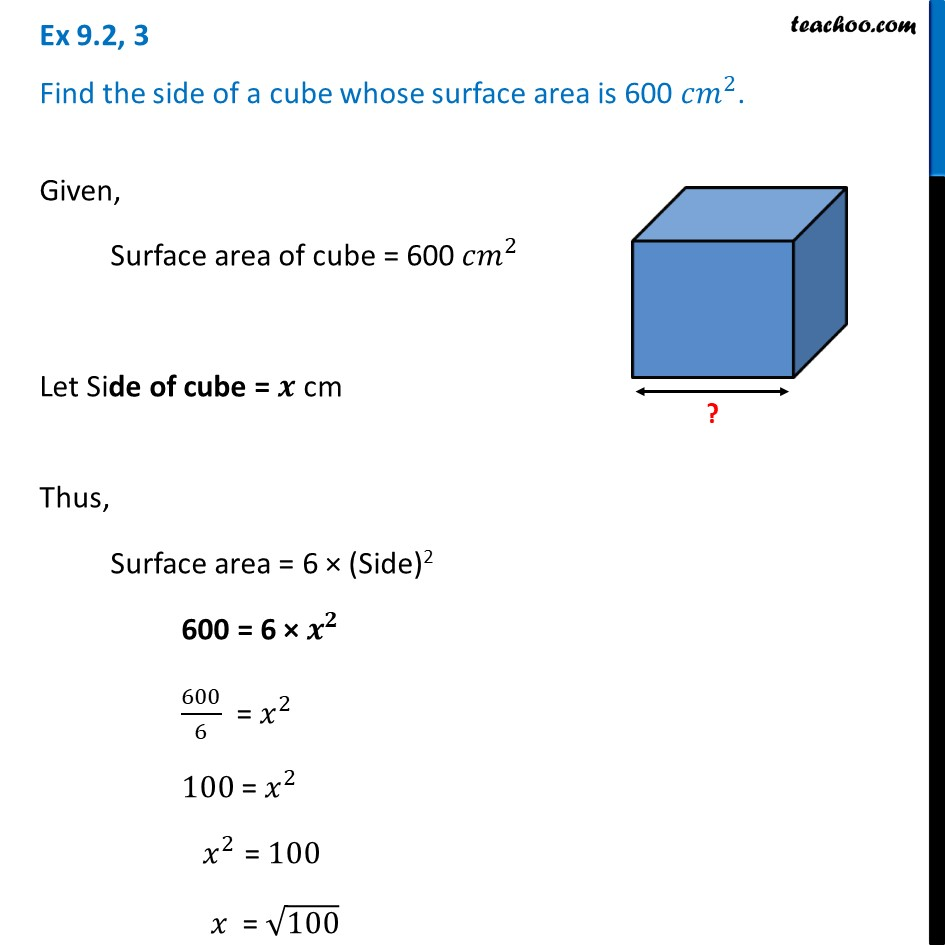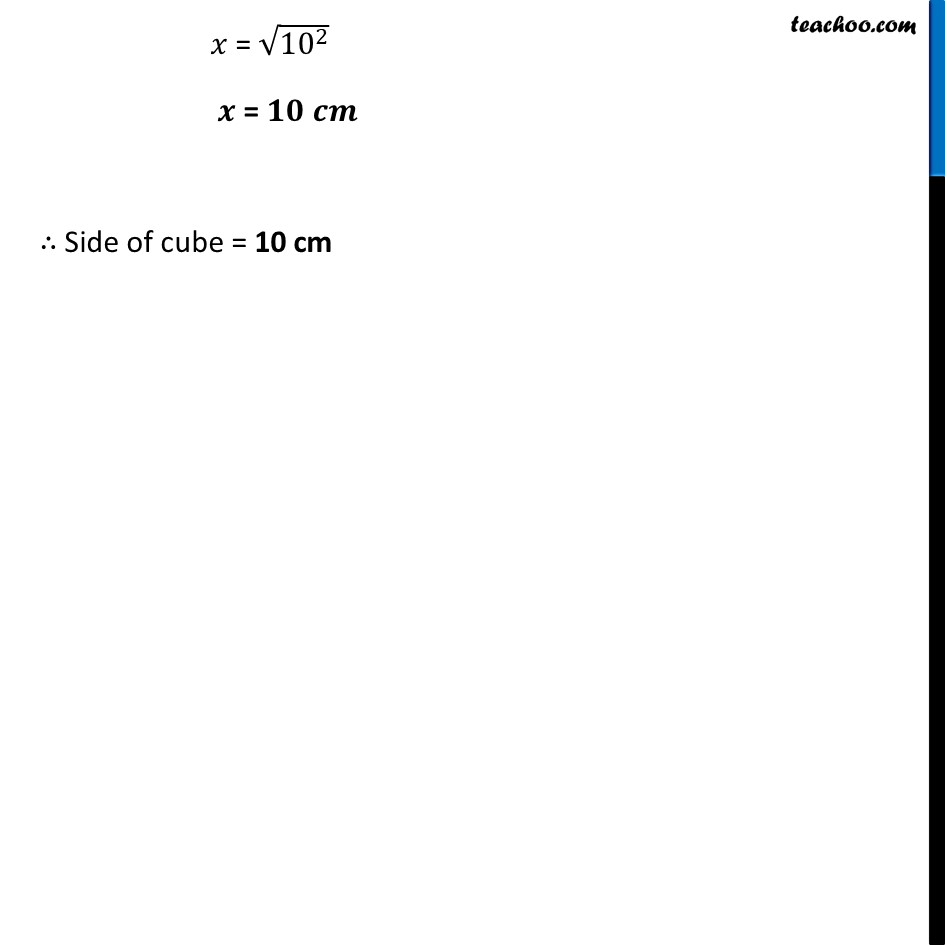Ex 9.2

Chapter 9 Class 8 Mensuration
Serial order wiseLearn in your speed, with individual attention - Teachoo Maths 1-on-1 Class

### Transcript

Ex 9.2, 3 Find the side of a cube whose surface area is 600 〖𝑐𝑚〗^2.Given, Surface area of cube = 600 〖𝑐𝑚〗^2 Let Side of cube = 𝒙 cm Thus, Surface area = 6 × (Side)2 600 = 6 × 𝒙^𝟐 600/6 = 𝑥^2 100 = 𝑥^2 𝑥^2 = 100 𝑥 = √100 𝑥 = √(10^2 ) 𝒙 = 𝟏𝟎 𝒄𝒎 ∴ Side of cube = 10 cm